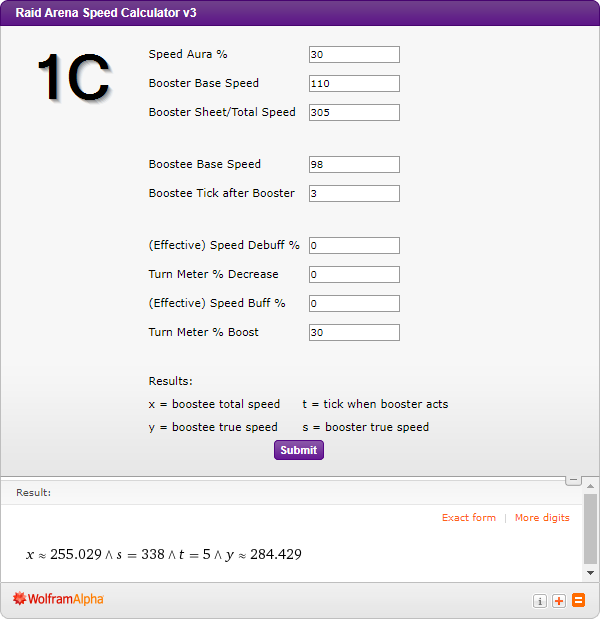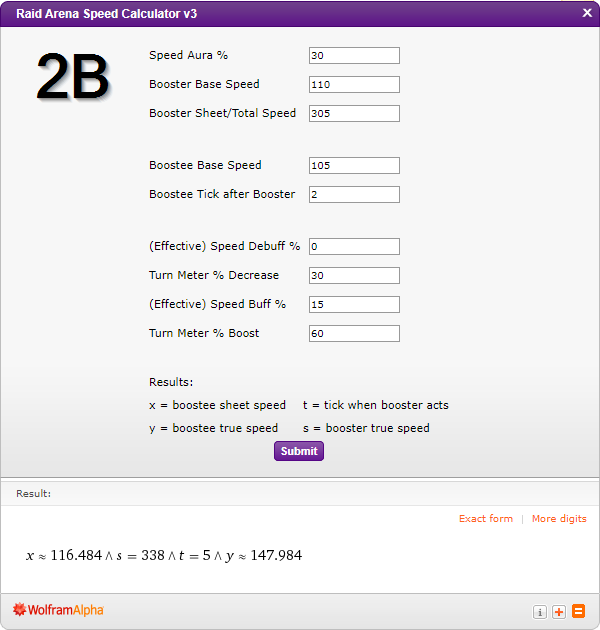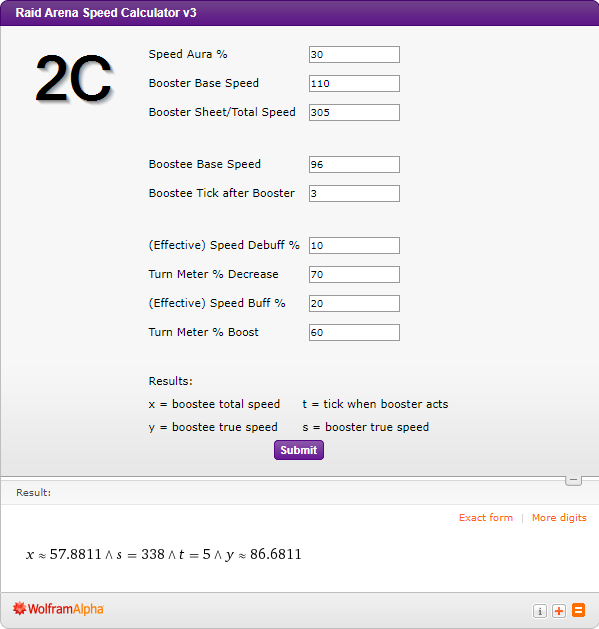## by Wystix

Outline

Introduction

In the current Raid arena meta, speed is king. The vast majority of compositions at high level arena include both a "speed lead" (i.e. champion providing a speed aura) and a "speed booster" (i.e. champion built with the very high speed, who can ensure your other champions act next through turn meter (TM) boosts and/or and speed buffs). One of the biggest sources of frustration for both new and experienced players building these compositions is when their speed booster wins the race to act first, but the opponent's speed booster "cuts in line" and manages to go second despite your champs receiving a turn meter boost and/or speed buff.  Preventing being cut is actually just a matter of ensuring that your second, third and fourth champions meet certain speed thresholds. This article will break down the math behind determining those speed thresholds and will also provide a calculator that can be used to determine the speed thresholds your champions need to meet.

Arena Algebra

The next section isn't intended to be a comprehensive review of speed mechanics, but a brief overview of the four key equations that go into calculating arena speed thresholds. If you're already familiar with arena speed math or not very interested in the details feel free to skip down to the calculator and examples below.

1. True Speed

• ​A champion's "true speed" or the speed that they actually act at in combat is a combination of their total/sheet speed (base speed, equipment, masteries) and is modified by the speed aura. It's given by y = x + a * b where = true speed, x = total/sheet speed, a = speed aura % and b = base speed.

• y = x + a * b

• The total/sheet speed x refers to speed displayed on your champion's stat page. It's important to know, however, that this number can be off the actual precise value by +/- 1 due to rounding errors. Usually, the displayed sheet speed is a close enough approximation for arena speed math, but if you want to find the most accurate value you can use the true speed calculator built into DeadWoodJedi's clan boss page to find the precise value.

2. Turn meter fill rate

• The most accurate equation for determining turn meter based on speed is Equation 2 where m = turn meter, y = true speed, i = ticks. Ticks are increased by 1 for all champions until someone has > 100 turn meter value. If multiple champions have > 100 turn meter, the champion with the highest turn meter has priority and acts first. If you want a more basic overview of how ticks and turn meter work, I recommend watching Plarium's video. Be aware, however, that there is one important detail in the video that is inaccurate. Champions definitely do benefit from a turn meter boost even if they already have >100 turn meter.

• m = 0.07 * y * i (2)

3. Tick on which our speed booster acts

• Because we know that champions act when their turn meter > 100 and ticks can only be integers, we can rewrite Equation 2 as Equation 3 where t is the tick on which our speed booster acts and the "Ceiling" function simply rounds up to the next whole number so we don't get impossible values of t.

• t = Ceiling[ 100/(0.07 * y) ]  (3)

4. Turn Meter Comparison

• This last equation is the core of figuring out how to not be cut by an opponent's booster. To derive it, we begin by assuming that our opponent's speed booster has a true speed (s) equal to our own booster's true speed, then we compare what the turn meter value would be for our opponent's booster at the tick when our first "boostee" needs to act. Our champion, however, has one or more advantages in this second tick speed race. Our speed booster can grant our following champions a turn meter boost % (o) and/or a speed buff % (f). The turn meter boost o will simply be added directly to our champion's turn meter value. The speed buff f will increase the amount of turn meter we gain from each successive tick. Putting this together we get Equation 4a.

• 0.07 * s * (t + 1) = 0.07 * y * (t + 1 * (1 + f/100)) + o (4a)

• It's important to note that Equation 4a only works for our first boostee. If we want to know the required speed for the champions that act 2 or 3 ticks after our booster we can add the variable *c* to the equation. If the first boostee also has an ability that provides a turn meter boost or a speed buff we simply sum the turn meter boosts for a new value of o and average the presence/absence of speed buffs for a new value of f that applies to the calculations for the subsequent boostees. This gives us Equation 4b.

• 0.07 * s * (t + c) = 0.07 * y * (t + c * (1 + f/100)) + o (4b)

• Finally, in addition to turn meter boosts and speed buffs for our champions, we can help ensure our champions take their turns next using turn meter decreases and speed debuffs. By adding the variables d for speed debuff % and e for turn meter decrease, we can get Equation 4c. Relying on these offensive turn meter abilities is risky, because Decrease Speed debuffs can be blocked by "Block Debuffs" and both can be resisted by the opposed Accuracy vs Resistance check. However, if these abilities do land they can be powerful tools in ensuring our champions get to take the critical next turns.

• 0.07 * s * (t + c * ( 1 - d/100)) - e = 0.07 * y * (t + c * (1 + f/100)) + o (4c)

Speed Calculator

Because all of the variables except our boostee's required sheet speed x are known quantities in the equations above, it is possible to use a series of substitutions to give us the horribly complex Equation #?\$! that combines everything into one "line" to calculate a required true speed:

• x = (0.07 * s * ( Ceiling[ 100/(0.07 * y) ] + c * ( 1 - d/100)) - (e + o )) / (0.07 * y * ( Ceiling[ 100/(0.07 * y) ] + c * (1 + f/100))) - a * s  (#?\$!)

Instead of using that horribly complex one line equation, we can instead use the system of equation solver functionality of Wolfram Alpha to present the data in a much more mathematically intuitive format. The default values for the calculator below represent the following scenario:

• Golden Reaper has a sheet/total speed of 280 and a base speed of 111.

• Golden Reaper uses her A3 Alacrity which places an 30% increase SPD buff on all allies and boosts their turn meter by 20%.

• The champion we want to go next (Skullcrown) has a base speed of 96 and provides a 24% speed aura.

ARENA CALCULATOR

Single Booster Example

As our first example, we'll calculate the speeds required not to be cut if our team has a 30% speed aura from our speed booster 1) Arbiter (305 sheet speed, 110 base speed) and we want our boostees to go in the following order: 2) Umbral Enchantress (base 98), 3) Venus (base 112), 4) Rae (base 98). We would enter the numbers into the calculator and get the results shown in Fig 1.Notice that speed requirements to not be cut is actually increasing as we move down our intended order of #2 Umbral Enchanctress (sheet: 237, true: 266), #3 Venus (sheet: 243, true: 276) and #4 Rae (sheet: 255, true: 284). This actually makes sense because as we move down our intended turn order we are giving our opponent's speed booster (true speed up to 338) additional ticks to make up for the one time 30% turn meter boost given by our Arbiter. If we truly want to make sure that our entire team moves before our opponent does, we will need to put our #4 Rae at her calculated sheet speed of 255 and ensure that #2 Umbral and #3 Venus both have true speed higher than Rae's 284 so they can still take their turns in the proper order.

Because of the challenge of getting our Rae's speed up to 255 while ensuring that she has enough crit rate/crit damage and accuracy to be an effective nuker, it may be a better idea to run Rae and possibly Venus at a much slower speeds. Instead, we can decide that only Umbral Enchantress needs to run at the speed where she can't be cut and give her the necessary accuracy to taunt the enemy team before they can use their powerful abilities.

Multiple Booster/Debooster Example

Because the speed requirements for a full set of 3 boostees to not be cut are generally so high, it is often advantageous to run two (or even more) boosters/deboosters. To provide an example on how to use the calculator for these advanced scenarios I'll use Arbiter (Sheet: 305, Base: 110 ) as the primary booster followed by #2 Lyssandra (Base 110) using her A3 (30% turn meter boost, 30% speed buff, 30% turn meter decrease), #3 Psylar (Base 105) using her A3 (30% speed debuff, Turn Meter Decrease 40%), and #4 Skullcrown (base 96) as the finisher.The calculation (Fig 2a) for Lyssandra's required speed is nearly identical to the previous example and gives 234 sheet and 267 true as the result. Calculating the required speed for #3 Psylar is more complicated. We begin by changing the Boostee Tick to 2 and then sum the turn meter boosts (30% + 30% = 60%) and turn meter decreases (0% + 30% = 30%) respectively. To properly input speed buff %, however, we need to figure out what the "effective speed buff" is over the two ticks that have happened so far. Psylar's turn meter has increased one tick at a 0% speed buff, and one tick at a 30% speed buff. Averaged over the two ticks that have happened so far we get a 15% "effective" speed buff that we enter into the calculator (Fig 2b) which gives us 116 sheet speed and 148 true speed as a result.

For the final calculation (Fig. 2c), we once again sum the turn meter boosts (30% + 30% + 0% = 60%) and turn meter decreases (0% + 30% + 40%= 70%). We also average the speed buffs ([0% + 30% + 30% ]/3 = 20%) and speed debuffs ([0% + 0% + 30% ]/3 = 10%) that have been active over the past three ticks. Entering #4 Skullcrown's base speed of 96 and the boostee tick value of 3 gives us the result of 58 sheet speed and 87 true speed. This essentially confirms that the combination of Arbiter, Lyssandra and Psylar provides nearly enough turn meter control to ensure that a pet rock will take its turn before the opponent's speed booster.

There are two important qualifications that apply to this final example calculation beyond the usual statement that opponents can resist turn meter decreases and speed debuffs. First, our calculator is actually only checking that the turn meter value for Skullcrown is higher than the opponent's speed booster at the start of the third tick after our booster. The substantial turn meter decreases that have been applied may have decreased the opponent's turn meter value below 100 which could mean that no one takes a turn on this particular tick if our #4 Skullcrown were somehow running at the impossibly slow 58 sheet speed.

The second caveat is that our substantial turn meter increases and speed buffs during the first three ticks may have boosted Arbiter's turn meter above 100 and above our Skullcrown's turn meter value, in which case Arbiter might take a second turn instead. Because these scenarios rely on an impossibly low equipped speed for Skullcrown figuring out which of these scenarios might apply and how to adjust the turn meter calculator to account for them will be left as an exercise for the reader.

Final Notes

While having a properly speed-tuned team is one of the best things you can do to ensure that you are properly executing your Arena strategy, speed calculators like the one I've presented here can't account for Champion passive abilities that manipulate and bypass turn meter as well as masteries that do so as well. Of particular note is the mastery Cycle of Revenge which increases the turn meter of a champion when an ally is hit by a critical attack. The complexities introduced by these abilities playing against each other is a big part of what I think makes Arena a fascinating and compelling game mode.